# Table of Content

• Important Definitions
• Homogeneous and Heterogeneous Data
• Entropy and Information Gain
• Gini and Gini Impurity
• Reduction in Standard Deviation
• Different Nodes in Decision Tree
• Different Algorithms used to build Decision Tree
• Decision Tree for Classification
• Building a decision tree for the given data using Entropy and Information Gain
• Building a decision tree for the given data using Gini and Gini Index
• Complete code to build decision tree in python
• Decision Tree for Regression
• Build Decision Tree using Standard Deviation Reduction strategy

# Important Definitions

## Homogeneous and Heterogeneous Data Nodes

Understanding homogeneous nature of data nodes is very important when it comes to decision tree. It is directly related to the predictive power of the tree build. If you pick a random data item from a fully homogeneous node then it wont be any brainier for you to tell which class it belongs to. Got the idea? if not then let me explain what is meant by homogeneous and non homogeneous data nodes.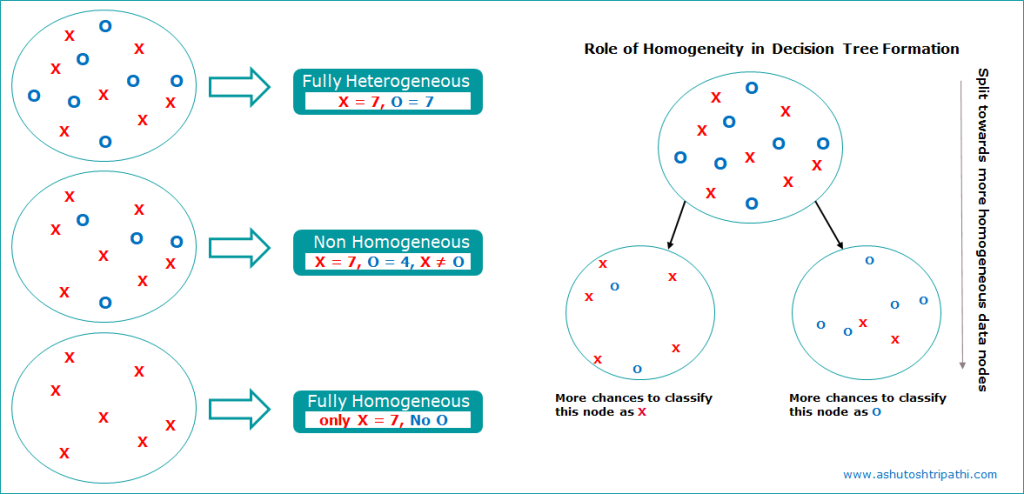Homogeneous and Heterogeneous Data node in Decision Tree | Source Website: www.ashutoshtripathi.com

## Entropy and information Gain

Entropy is the measure of randomness in your data. More randomness means more entropy means harder to draw any conclusion or harder to classify. Lets relate it to the homogeneity concept explained above to make it easy to understand. If your data node is heterogeneous it will be hard to draw any conclusion about that data node which will lead to higher entropy.

## Entropy Calculation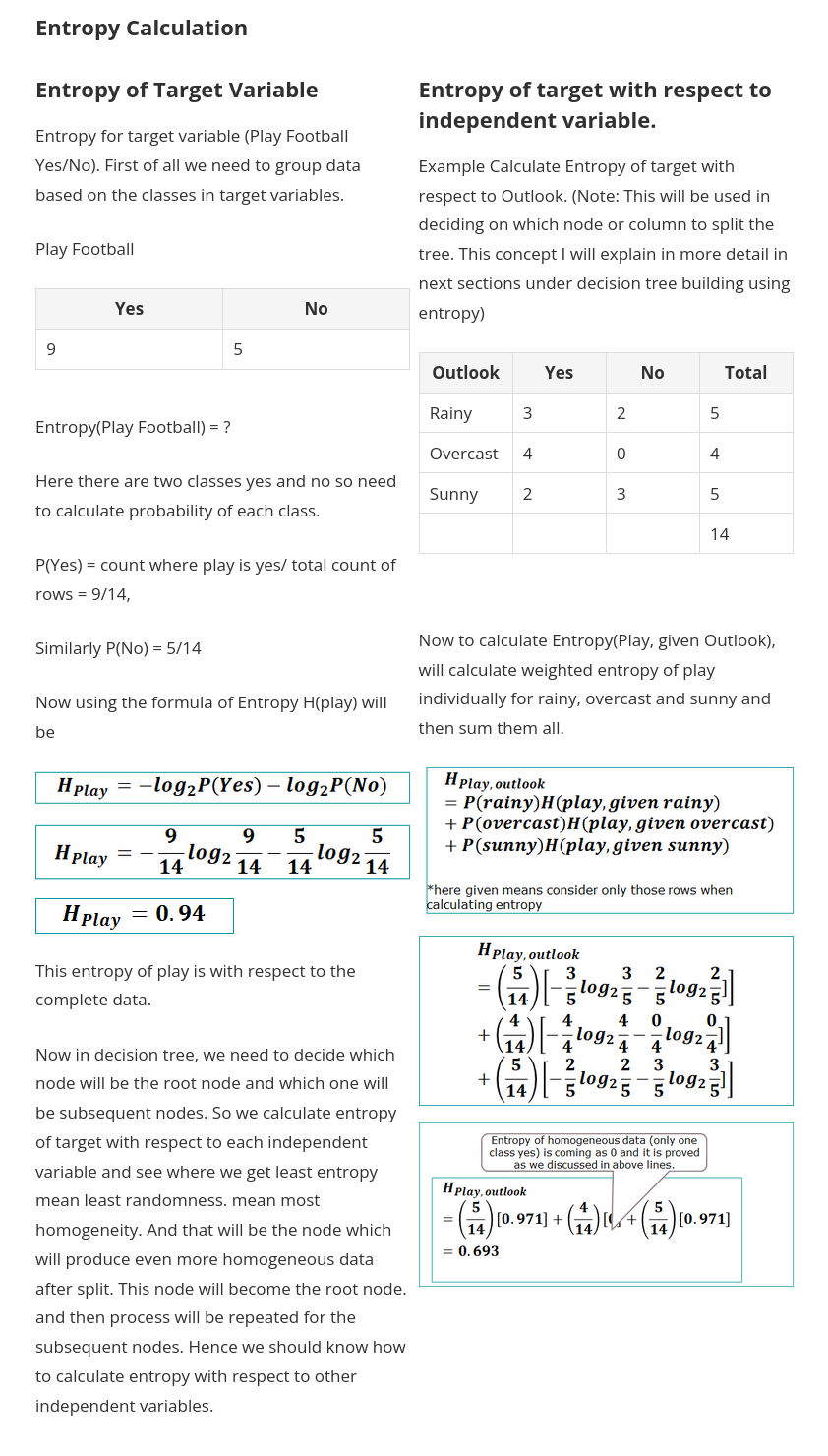Entropy Calculation for different data nodes in Decision Tree | Source : www.ashutoshtripathi.com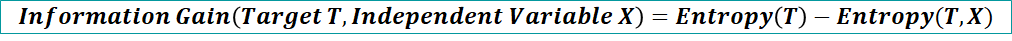Information Gain Formula in Decision Tree | source: www.ashutoshtripathi.com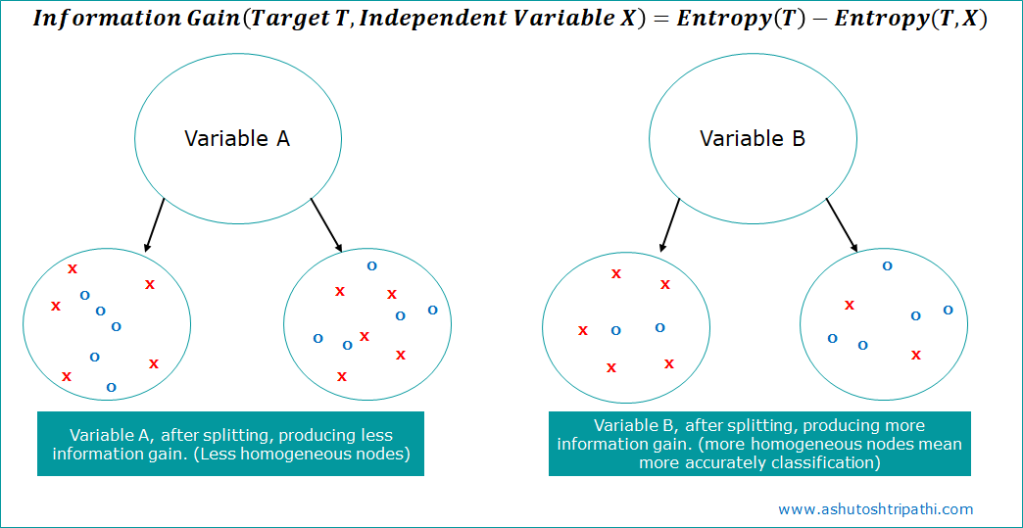Information Gain Info graphic Decision Tree | Source: www.ashutoshtripathi.com

## Gini and Gini Impurity

Gini is calculated by using the formula — the sum of square of probability for success and failure (p²+q²)Gini Formula in Decision Tree | Source: www.ashutoshtripathi.com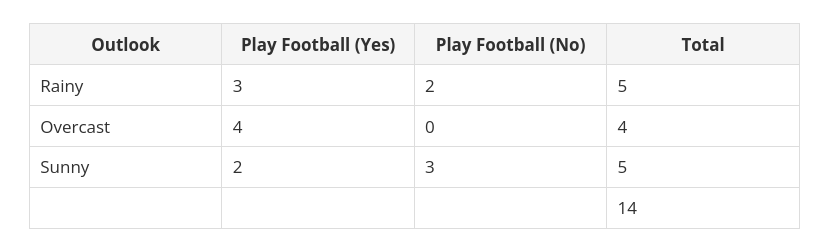Gini calculation for outlook column in decision tree | source: www.ashutoshtripathi.com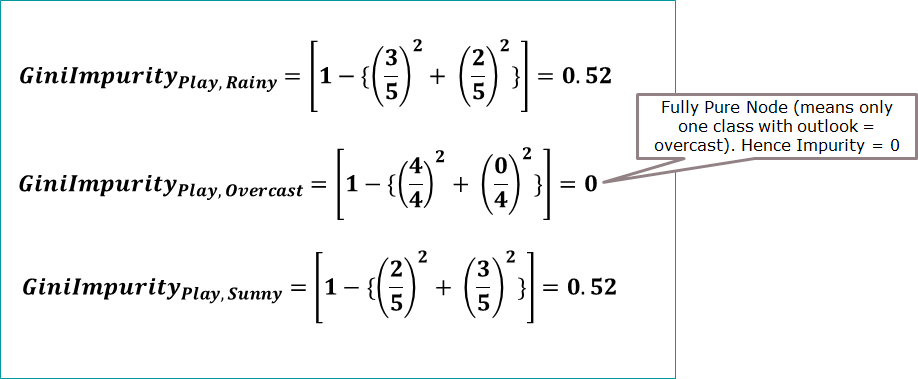Gini Impurity calculation example | source: www.ashutoshtripathi.com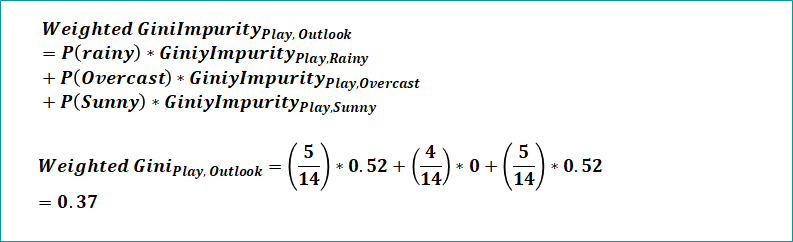weighted gini impurity in decision tree example | source: www.ashutoshtripathi.com

# Reduction in Variance or Standard Deviation Reduction — SDR

Entropy, Information Gain and Gini Impurity are used in classification problems. So what about regression? So similar to information gain that is reduction in entropy, we have reduction in variance or reduction in standard deviation which is used for regression type problem in decision tree.

# Understand different nodes in Decision Trees

1. Root Node: It represents the entire population or sample and this further gets divided into two or more homogeneous sets. If you consider Information gain then the node which has maximum information gain will become the root node.
2. Decision Node: When a sub-node splits into further sub-nodes, then it is called the decision node. Basically it helps you to decide whether to choose (select or go) right sub tree or left sub tree
3. Leaf / Terminal Node: Nodes do not split is called Leaf or Terminal node.
4. Branch / Sub-Tree: A subsection of the entire tree is called branch or sub-tree.
5. Parent and Child Node: A node, which is divided into sub-nodes is called a parent node of sub-nodes whereas sub-nodes are the child of a parent node.
6. Pruning: When we remove sub-nodes of a decision node, this process is called pruning. You can say the opposite process of splitting. It is used in hyper parameter tuning in decision trees.

# Different Algorithms used to build Decision Tree

Decision Tree is used for both types of problems Classification and Regression. So based on problem types it uses different algorithms.

# Decision Tree for Classification Problems

## Building a decision tree for the given data using Entropy and Information

Please watch the video explaining decision tree formation and split using entropy and information gain.

## Building a decision tree for the given data using Gini and Gini Index

Please watch the video which explains decision tree formation using gini and gini index.

# Complete Code to build Decision Tree for classification using Python

Please refer my post on step by step guide to build decision tree using python.

# Decision Tree for Regression

Please refer my post on step by step guide to build decision tree for Regression

--

--